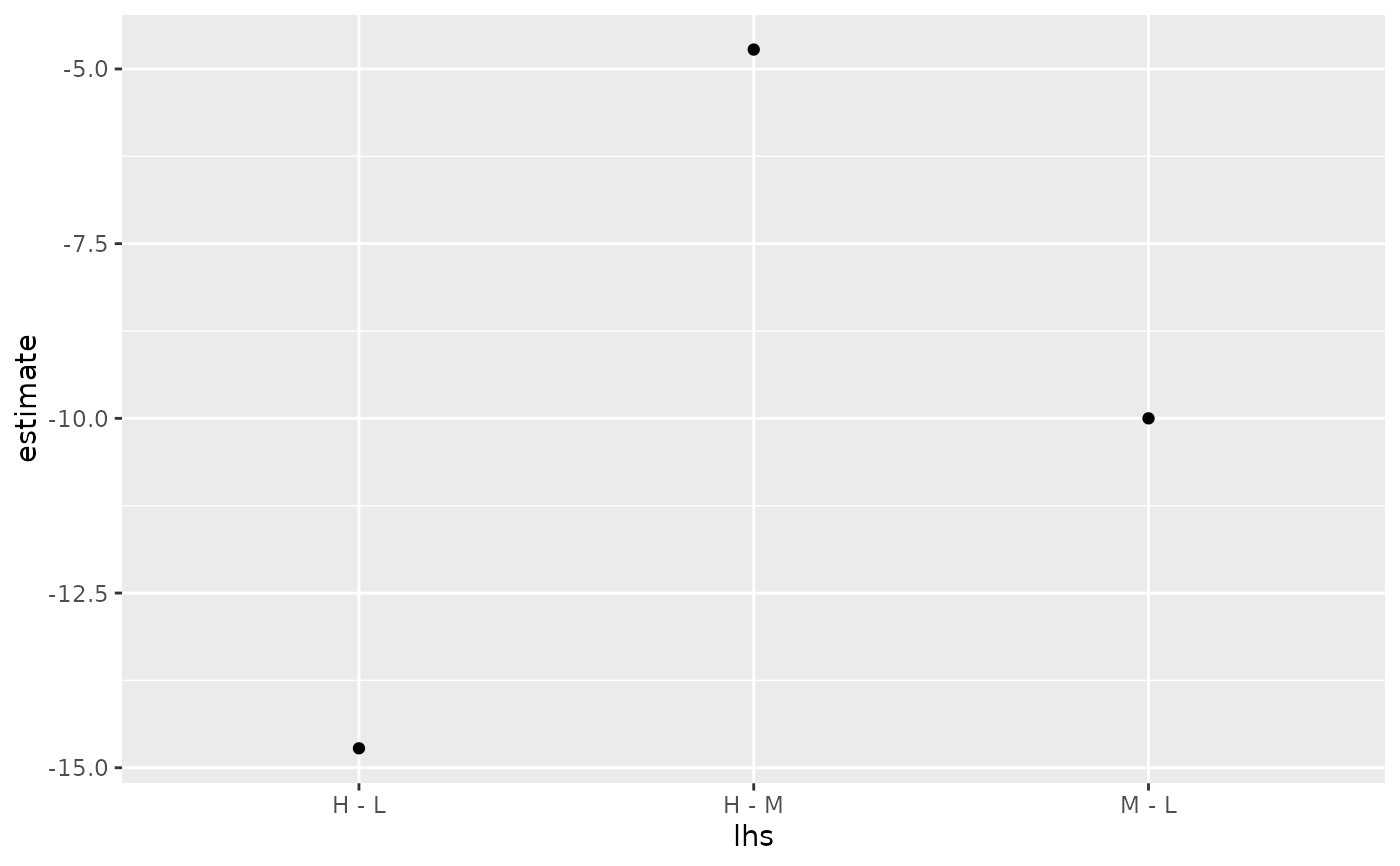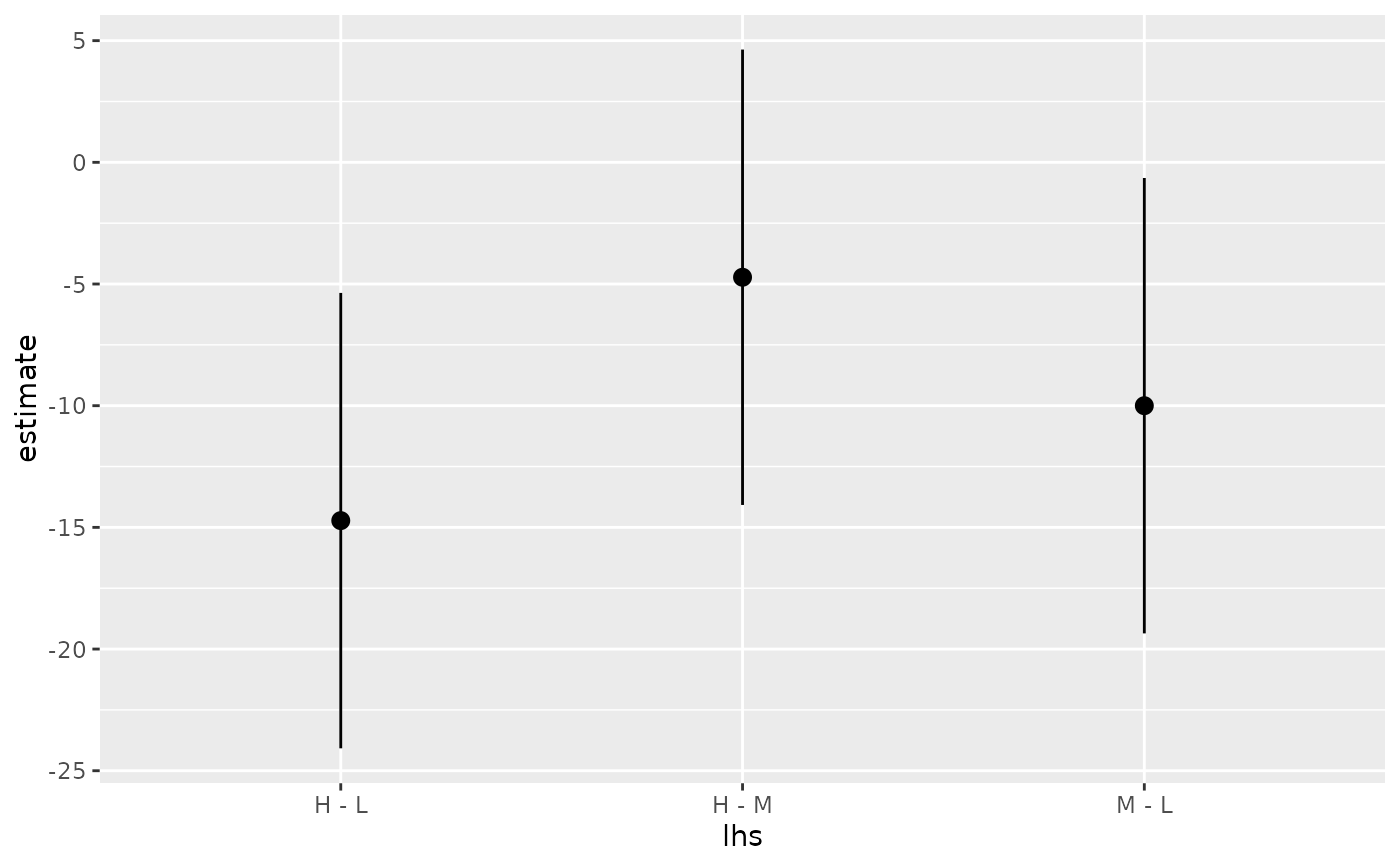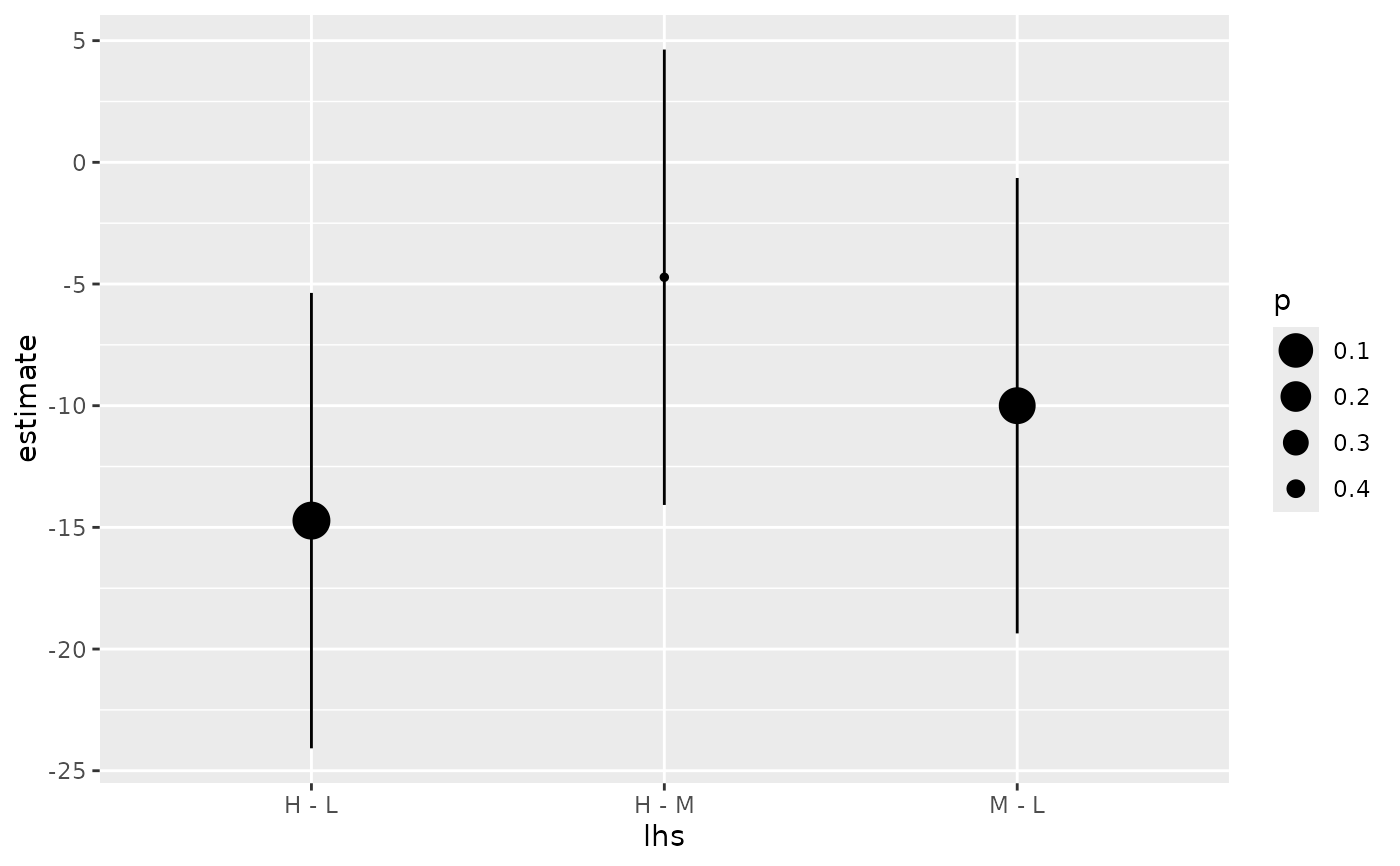Tidy summarizes information about the components of a model. A model component might be a single term in a regression, a single hypothesis, a cluster, or a class. Exactly what tidy considers to be a model component varies across models but is usually self-evident. If a model has several distinct types of components, you will need to specify which components to return.

## Usage

# S3 method for summary.glht
tidy(x, ...)

## Arguments

x

A summary.glht object created by calling multcomp::summary.glht() on a glht object created with multcomp::glht().

...

Additional arguments. Not used. Needed to match generic signature only. Cautionary note: Misspelled arguments will be absorbed in ..., where they will be ignored. If the misspelled argument has a default value, the default value will be used. For example, if you pass conf.lvel = 0.9, all computation will proceed using conf.level = 0.95. Two exceptions here are:

• tidy() methods will warn when supplied an exponentiate argument if it will be ignored.

• augment() methods will warn when supplied a newdata argument if it will be ignored.

tidy(), multcomp::summary.glht(), multcomp::glht()

Other multcomp tidiers: tidy.cld(), tidy.confint.glht(), tidy.glht()

## Value

A tibble::tibble() with columns:

contrast

Levels being compared.

estimate

The estimated value of the regression term.

null.value

Value to which the estimate is compared.

p.value

The two-sided p-value associated with the observed statistic.

statistic

The value of a T-statistic to use in a hypothesis that the regression term is non-zero.

std.error

The standard error of the regression term.

## Examples


# load libraries for models and data
library(multcomp)
library(ggplot2)

amod <- aov(breaks ~ wool + tension, data = warpbreaks)
wht <- glht(amod, linfct = mcp(tension = "Tukey"))

tidy(wht)
#> # A tibble: 3 × 7
#>   term    contrast null.value estimate std.error statistic adj.p.value
#>   <chr>   <chr>         <dbl>    <dbl>     <dbl>     <dbl>       <dbl>
#> 1 tension M - L             0   -10         3.87     -2.58     0.0337
#> 2 tension H - L             0   -14.7       3.87     -3.80     0.00109
#> 3 tension H - M             0    -4.72      3.87     -1.22     0.447

ggplot(wht, aes(lhs, estimate)) +
geom_point()CI <- confint(wht)

tidy(CI)
#> # A tibble: 3 × 5
#>   term    contrast estimate conf.low conf.high
#>   <chr>   <chr>       <dbl>    <dbl>     <dbl>
#> 1 tension M - L      -10       -19.4    -0.643
#> 2 tension H - L      -14.7     -24.1    -5.37
#> 3 tension H - M       -4.72    -14.1     4.63

ggplot(CI, aes(lhs, estimate, ymin = lwr, ymax = upr)) +
geom_pointrange()tidy(summary(wht))
#> # A tibble: 3 × 7
#>   term    contrast null.value estimate std.error statistic adj.p.value
#>   <chr>   <chr>         <dbl>    <dbl>     <dbl>     <dbl>       <dbl>
#> 1 tension M - L             0   -10         3.87     -2.58     0.0336
#> 2 tension H - L             0   -14.7       3.87     -3.80     0.00121
#> 3 tension H - M             0    -4.72      3.87     -1.22     0.447
ggplot(mapping = aes(lhs, estimate)) +
geom_linerange(aes(ymin = lwr, ymax = upr), data = CI) +
geom_point(aes(size = p), data = summary(wht)) +
scale_size(trans = "reverse")cld <- cld(wht)
tidy(cld)
#> # A tibble: 3 × 2
#>   tension letters
#>   <chr>   <chr>
#> 1 L       a
#> 2 M       b
#> 3 H       b